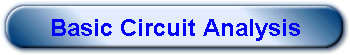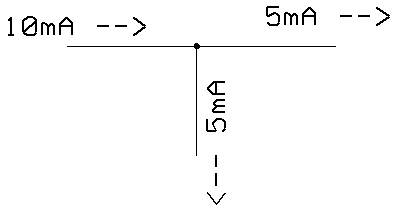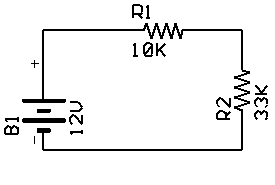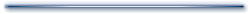From time to time, questions arise on various forums about how to calculate the dropping value of a resistor, or design a voltage divider. You'd be amazed at how simple these things are if you understand three basic laws:

1. Ohm's law

Ohm's law says that the voltage (E) across a resistor (R) will be the produce of the resistance times the current (I). In other words E=I*R. Using algebra you can deduce then that I=E/R and R=E/I. So if you know any two of these three values, you can get the third one.

2. Kirchoff's Current Law

This is simple. If you have a bunch a wires that come together at a point, all the currents going in must equal all the current going out. In your circuit you usually write current going one way as positive and the other way as negative, so another way of saying this is that adding up all the current must  result in 0. This is common sense, really. Say you have two light bulbs connected to a battery in parallel. Each bulb is known to draw 200mA. How much current is the battery supplying? Of course, 400mA. That's Kirchoff's law in action. If each bulb draws 200mA, the battery must supply 400mA to light them both. Another application of this law is that in a series circuit, the current is the same throughout. That's because the current going through one component, must be the same as the current going through the other component so that the sum of currents can be 0. So if you have an LED and a resistor in series with a battery, and someone tells you the resistor has 10mA through it, the LED must also have 10mA going through it. Notice this isn't the same as saying the LED's maximum current is 10mA, therefore the circuit must have 10mA in it. That would be like saying, the car's speedometer goes to 200KPH, so that's how fast we must be going! It is, however, like saying, our speedometer says 90KPH and the car next to us looks like it is not moving, so it must be going 90KPH too.3. Kirchoff's Voltage Law

Another aspect to Kirchoff's law is that all the voltage drops in a loop must also equal zero. For the purposes of this discussion, we can assume that this means circuits in parallel have the same voltage across them. Going back to my 200mA light bulb example. They are both connected to the + and - lead of the battery, so they are in parallel. It is not possible that one light bulb sees 12V while the other sees 10V (assuming no circuit defects, like a resistive wire or some other fault). Both bulbs will see the same voltage.LEDs

So the common problem is you have an LED that is rated at 1.2V @ 10mA. You want to use a 5V supply. What is the value of the dropping resistor? By applying Kirchoff's voltage law (#3) you know that if the LED is going to drop 1.2V, the remaining voltage is 5-1.2 or 3.8V. Law #2 tells you that if you want 10mA to flow through the LED, you will also have to have 10mA flowing through the resistor. So now you know that the resistor has to drop 3.8V at 10mA. Applying Ohm's law, you can see that 3.8/.01 = 380 or 380 ohms. Of course, if you come up with a non-standard value, you'll have to pick one that is close. For example, suppose the closest value you have is 470 ohms. The LED always drops 1.2V, so the resistor always drops 3.8V. 3.8V/470 (law #1) = about 8mA. The LED won't be as bright, but it should still be fine.Try these:

LED is 2V @ 15mA and a 12V supply (click for answer)

LED is 1.4V @ 5mA and a 3V supply (click for answer)

Voltage Dividers

What about voltage dividers? This is the classic network where you hook two resistors in series with a battery. The center point of the resistors develops a lower voltage than the battery. When you hook a pot up across a battery, you are creating a voltage divider where the pot's wiper is the center of the divider network. If the resistors are equal, the voltage at the center will be half the battery voltage.

That's easy to remember, but what if the resistors are different values? Suppose the resistor is connected to the + terminal is 10K (R1) and the - terminal has a 33K resistor (R2) and they meet in the middle (assume a 12V battery). Simple application of the three laws will let you easily analyze this situation. Two observations make this circuit simple. First, the resistors are in series, so they must have the same current flowing in them (law #2). Second, the voltage across each resistor must add up to equal the battery voltage (law #3).What's the current? Resistors in series add up, so the total resistance is 43K. Applying Ohm's law, you can see that 12/43K = .279mA or 279uA. Both resistors have this current flowing through them. Therefore, it is easy to calculate the voltage across both. The "output" voltage will be the voltage across R2 or 33K * 279uA = 9.2V (about). Yes, you can get the same answer by memorizing that:

Vo = Vin*R2/(R1+R2)

But look at that computation. What is it? Rewrite it a little and you have Vo = R2 * Vin/(R1+R2) which is just what we did. R1+R2 is the total resistance. Vin divided by the total resistance is the current. And R2 times the current is the answer.

Voltage dividers may not seem very important on the face of it, but many more complex circuits are voltage dividers or can be modeled by voltage dividers. For example, a Wheatstone bridge is just two voltage dividers, side by side. Op amp feedback circuits are often voltage dividers, as is the base biasing network of a common emitter amplifier.

Try these dividers:

Vin=12V R1=10K R2=20K (click for answer)

Vin=9V R1=1K R2=200 (click for answer)

Practical Voltage Division

Don't fall prey to the temptation to use a voltage divider instead of a voltage regulator. Voltage dividers are totally dependent on their supply voltage, so don't try to "regulate" 12V to 5 with a divider. However, there are cases where you might want to use a divider. For example, perhaps you have a 5V A/D converter, but you need to measure 0-10V with it. Of course, you will lose precision, but maybe that's OK.

Converting 10V to 5V is easy, right? Two 10K resistors and you have a 50% voltage divider. Except it isn't that simple. If you build a voltage divider in the lab, you'll look at the output with a voltmeter or a scope. All modern DVMs have input impedances of 10M or more, so for practical purposes it isn't there as far as the circuit is concerned. But when you draw real power from the center node, you are effectively putting another resistor in parallel with R2. This changes the circuit and the output voltage.

Suppose your A/D convert has an input resistance of 25K. Now you don't have two 10K resistors. You have R1=10K and R2=10K and 25K in parallel. Resistors in parallel add their admittance, so:

Rtotal = 1/(1/R1+1/R2+.....1/Rn)

If you only have two resistors, this can be simplified to: Rtotal = R1*R2/(R1+R2). So in the above example R2 is effectively 7143 ohms. The divider's ratio is then about 42% (7143/17143). Not the 50% you were looking for. This even happens with a meter, but the effect is so small you don't care. Consider if R2 was equal to 10K and 10M in parallel. That's 9990 ohms and the ratio is 49.97% -- hardly any difference at all, and probably less error than the 5% or 10% resistors you are using.

So when calculating your A/D converter input divider, you want to make R2's total value 10K. If the input resistance is 25K, you have to solve for the parallel resistor with 25K that gives you 10K. So:

10K = 25K*Rx/(25K+Rx)

A little algebra results in:

250K/15K = Rx or Rx=16.667K (you can verify that by computing 16.667K in parallel with 25K).

So the actual voltage divider would be R1=10K, R2=16.7K, but you can't really find that value more than likely. Substitute a 15K resistor and you'll find the true division ratio is just over 48% (can you get the same result?). However, 16K is a standard value and provides a ratio of 49.4% which is pretty close.

Thevinin's Equivalent

Another implication of voltage dividers is Thevinin's theorem. This states that any voltage source and an arbitrary network can be modeled by a voltage source and a voltage divider (just like the diagram above if you consider B1 as an ideal voltage source instead of a practical battery). The R1 part of the divider represents the bulk of the circuit. The R2 part is the "load" -- the part that consumes the output. Consider the above A/D example. The load is a 25K resistor that represents the A/D converter. The source is a 10V battery (the input). In between we have R1=10K and R2=16K.

To create the Thevinin equivalent, you consider what the voltage would be with no load at all. In this case it would be 10V through a 10K/16K voltage divider or 6.15V. That's the new voltage source. Next, pretend like the original voltage source was a short circuit and determine how much resistance is out the output terminals. In this case, shorting the battery makes R1 and R2 appear in parallel at the load terminals. That's the Thevinin resistance (6.15K).

So the equivalent circuit for analysis is a 6.15V battery with a 6.15K resistor in series with the + lead. The load connects between the far end of this resistor and the battery - lead. Guess what? That forms a voltage divider with R1=6.15K and R2=25K (the load). So what voltage will the load see (remember, even thought the battery is at 6.15V, it represents a 10V input)? The output will be 6.15V reduced by the divider or about 4.94V. How much current does the load consume? 4.94/25K or 197uA.

In this simple case, it was just as easy to analyze the network directly, but any large network can be reduced to a voltage divider with the same techniques. To summarize:

1. Remove the load from the circuit.

2. Determine the open circuit voltage at the load's connection point.

3. Pretend to short the voltage source

4. Compute the resistance between the load terminals.

5. Draw the Thevinin's equivalent using the voltage from Step 2 and the resistance from Step 4.

For a more complex example, consider a "T" circuit. The left hand branch of the T (R1) connects to a 10V battery. The bottom middle of the T (R2) connects to ground and the right hand part of the T (R3) connects to the load. Each resistor in the T is 10K. The open circuit voltage will be 5V. That's because R1 and R2 form a 50% voltage divider. R3 has no current flowing through it (it is an open circuit) and so it does not drop any voltage (R*0 is still 0). With the battery shorted, the effective resistance is 10K (R3) + 10K in parallel with 10K (R1 and R2). That's a total of 15K. So the equivalent circuit is a 5V battery with a 15K series resistor. Try computing the voltage delivered to a 50 ohm load without creating a Thevinin model. Then go back and do it with the Thevinin model -- much easier (Click here for the answer).

Speaking of 50 ohms, the Thevinin model can help you understand why matching antennas to transmitters is so important. Remember, power is voltage time current. Suppose your transmitter looks like a 10V battery with a 50 ohm resistor (the source impedance). Do the calculations for a 20 ohm load, a 50 ohm load, and a 100 ohm load:

 Load Voltage Current   (mA) Power (mW) 20 2.9 145 421 50 5.0 100 500 100 6.7 67 449

Higher load resistance makes more voltage, but less current. Lower resistance makes more current but less voltage. This is an effect of conservation of energy. The power maximum is at the point where the load and the source are equal. This is why you want 8 ohm speakers on an 8 ohm amplifier output, or a 50 ohm antenna on a transmitter.

If you have a 100W ham transmitter that should deliver to a 50 ohm load, what's the voltage presented to the load? Hint, if Power is E*I you can use Ohm's law to rearrange the equation:

P=EI
P=I*R*I = I**2 R (where I**2 is I squared)
P=E*E/R = E**2/R

To deliver 100W into a 50 ohm load, you have to find E**2 so that E**2/50 = 100. Therefore E**2 is 5000 and the square root of 5000 is 70.7V. The current will be 1.4A.

This explains why mismatched antennas cause transistor finals to fold power back (or blow up in the old days). Assume the transmitter is also a 50 ohm source impedance. That means it's Thevenin voltage is about 140V. Now replace the 50 ohm load with a 10 ohm load. What does the current rise to? (answer) How about the voltage across the load? (answer) That means the source resistor has to drop the balance (nearly 117V).